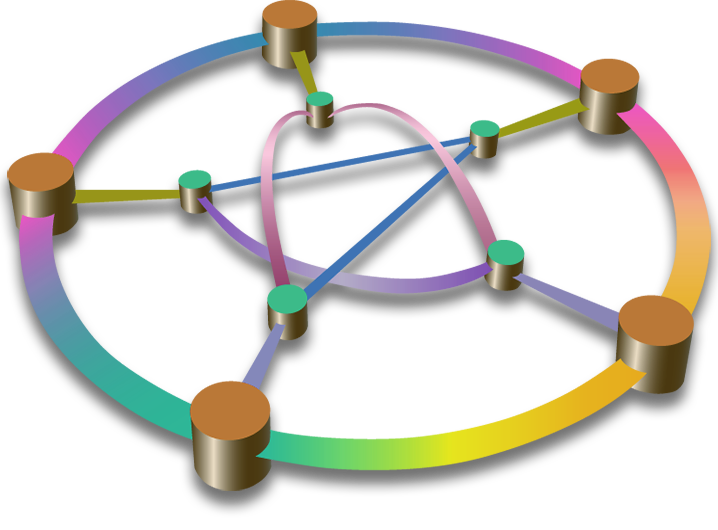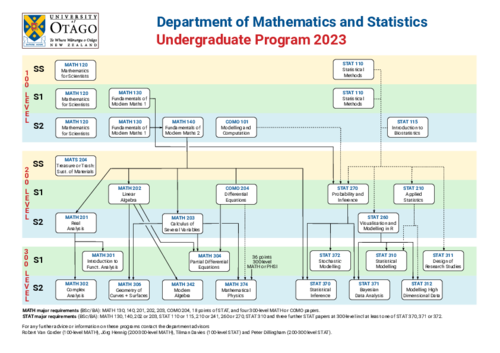Mathematics
Te Tari Pāngarau me te Tatauranga
Department of Mathematics & Statistics

## PapersStruggling to decide which 100-level Mathematics paper to take first?   See this discussion

The Department offers 16 Mathematics undergraduate papers at 100, 200 and 300-level (as well as a selection of 400-level postgraduate papers). This section includes short descriptions of these papers, together with links to individual illustrated paper pages which feature information about the subject, the paper and assessment procedures.

See the flowchart of available papers for 2023, their prerequisites and semesters.

Click the paper name below for complete details.

## 100 level

COMO101 Modelling and Computation     18 points   Second Semester

An introduction to mathematical modelling and associated scientific computation, with applications across a wide range of topics in every-day life, science, engineering, biomedicine, and business.

MATH120 Mathematics for Scientists     18 points   First Semester, Second Semester, Summer School

The modern world is built on science and technology. As such, the increasingly competitive job market requires new graduates to have confidence and fluency in quantitative problem solving. MATH120 uses a problem-based learning approach to develop problem-solving and critical thinking skills by consistently working through applied examples from a range of scientific disciplines, while learning new mathematical techniques and tools. Students will be encouraged to try different approaches, critically analyse their findings and communicate them orally and/or as written reports. As a result, MATH120 students will be ideally equipped to specialise in any discipline that includes a quantitative component.

MATH130 Fundamentals of Modern Mathematics 1     18 points   First Semester, Second Semester

The aim of MATH 130 is to develop confidence and capability in the concepts and techniques of modern mathematics and its applications. It provides background skills needed throughout the quantitative sciences and prepares you for further mathematical study.

The paper delivers a standard syllabus combining calculus and linear algebra, but does so in a way that demonstrates how different concepts link together and why they are important. Calculus has been described as one of the most important tools in science. It allows us to think mathematically about continuous change, area and volumes. Linear algebra is invaluable for working with processes and models in high dimensions, linking both with calculus as well as geometry and data science.

The calculus and linear algebra covered in this paper provide the foundation for future study in mathematics and also the application of mathematics in other disciplines.

MATH140 Fundamentals of Modern Mathematics 2     18 points   Second Semester

The techniques covered in this paper form the basic tools used to produce mathematical frameworks for modelling quantifiable phenomena. For example, to model the movement of an object through space, we begin with an algebraic structure in which to specify where our object is, and then study how that position changes with time using methods developed in calculus. Many other problems arising in areas such as Economics or Chemistry can be examined mathematically using the same basic principles. For example, we may need to minimise a manufacturing cost, or the time for a chemical reaction to take place, or the effects of river pollution; in each case the techniques used for the minimisation are based on a mixture of tools relying on both algebra and calculus.

This paper aims to develop proficiency with algebra and calculus, both for use in other subjects and in preparation for further study of Mathematics. MATH 140 is the natural continuation of MATH 130, and provides a strong mathematical background to support other subjects as well as forming a necessary prerequisite for progression to 200-level Mathematics.

## 200 level

COMO204 Differential Equations     18 points   First Semester

This course is an introduction to mathematical techniques useful for solving problems arising in the physical, health and life sciences, and commerce. Topics include analytical solutions of ordinary differential equations, Laplace transforms, systems of linear ordinary differential equations, and nonlinear dynamical systems.

MATH201 Real Analysis     18 points   Second Semester

MATH 201 is an introduction to the basic techniques of real analysis in the familiar context of single-variable calculus. This paper is compulsory for the Mathematics major.

MATH202 Linear Algebra     18 points   First Semester

MATH 202 is an introduction to the fundamental ideas and techniques of linear algebra, and the application of these ideas to computer science, the sciences and engineering. This paper is compulsory for the Mathematics major.

MATH203 Calculus of Several Variables     18 points   Second Semester

This paper is an introduction to the mathematics of curves, surfaces and volumes in three-dimensional space, and extends the notions of differentiation and integration to higher dimensions. It is a prerequisite for three level-300 MATH papers.

MATS204 Treasure or Trash: Sustainability of Materials     18 points   Summer School

Introduction

This paper develops understanding of the principles and complexities of sustainability of materials and their application in design, selection, processing, manufacture, use and disposal of products.

## 300 level

COMO303 Numerical Methods     18 points   First Semester
Not available in 2023

This paper develops the theory and techniques required to apply computational methods in modelling, applied mathematics and data analysis. Topics include matrix computation, data fitting, and the numerical solution of differential equations.

MATH301 Hilbert Spaces     18 points   First Semester

This paper is an introduction to Hilbert spaces and linear operators on Hilbert spaces. It extends the techniques of linear algebra and real analysis to study problems of an intrinsically infinite-dimensional nature.

MATH302 Complex Analysis     18 points   Second Semester

This paper develops the differential and integral calculus of functions of a complex variable, and its applications.

MATH304 Partial Differential Equations     18 points   First Semester

This paper gives an introduction to the theory of partial differential equations by discussing the main examples (Laplace's equation, Poisson's equation, transport equation, wave equation) and their applications.

MATH306 Geometry of Curves and Surfaces     18 points   Second Semester

This paper is an introduction to differential geometry; its focus is the structure of two-dimensional surfaces.

MATH342 Modern Algebra     18 points   Second Semester

This paper introduces groups and rings. These are algebraic structures consisting of a set with one or more binary operations on that set satisfying certain conditions. These structures are ubiquitous throughout modern mathematics and this paper examines their properties and some applications.

MATH374 Mathematical Physics     18 points   Second Semester

This paper presents the foundation theory for two major topics in Physics. The Classical Mechanics section introduces the formal framework of Classical Mechanics and illustrates its application to two-body problems, rotating systems, collisions, and chaos. The Special Relativity and Cosmology section covers the special theory of relativity with applications to relativistic mechanics, electrodynamics in covariant form, and cosmology.

This paper is the same as the PHSI336 paper offered by the Physics Department. It is taught jointly by staff from both Departments.